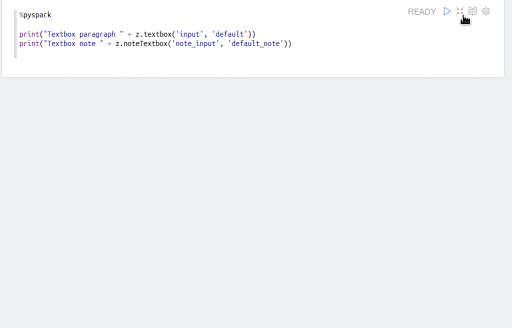# Using Dynamic Input Forms in Notebooks¶

Qubole supports Apache Zeppelin’s dynamic input forms and also based on the language backend. You can run the paragraphs several time after you change the input values. You can create forms by:

Dynamic forms are supported on Spark notebooks. For more information about parameterized notebook API that uses these dynamic forms to populate parameter values, see Run a Notebook.

## Using Form Templates¶

You can create a text-input form, select form, and a check box form using form templates. You can change the values in the input fields and rerun the paragraph as many times as required.

A simple form can be created as shown in this example.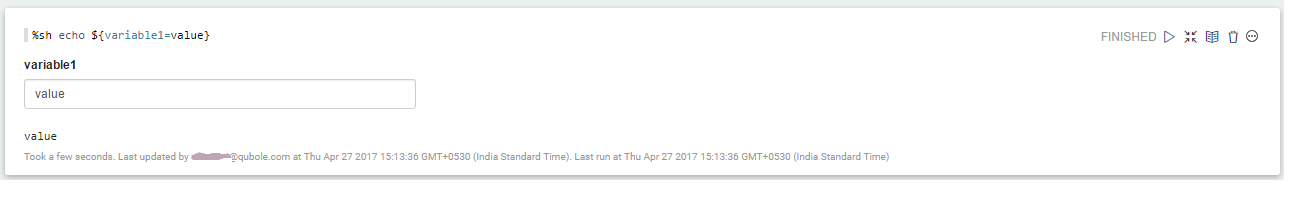A simple check box form can be created as shown in this example.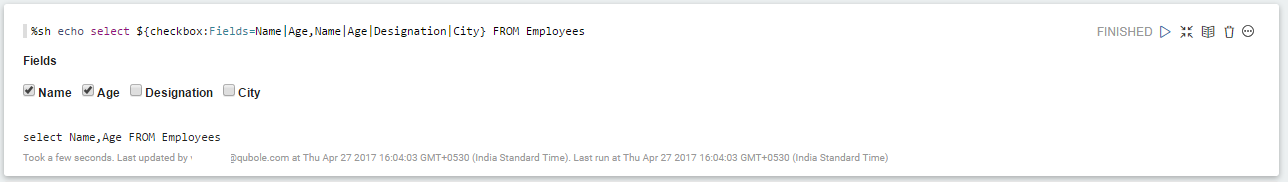## Using Programming Language¶

You can create a text input form, check box form using the scala (`%spark`) and Python (`%pyspark`) interpreters. As in using form templates, by using the programming language, you can also change the value of inputs and rerun the paragraphs as many times as required.

A simple form can be created as shown in this example.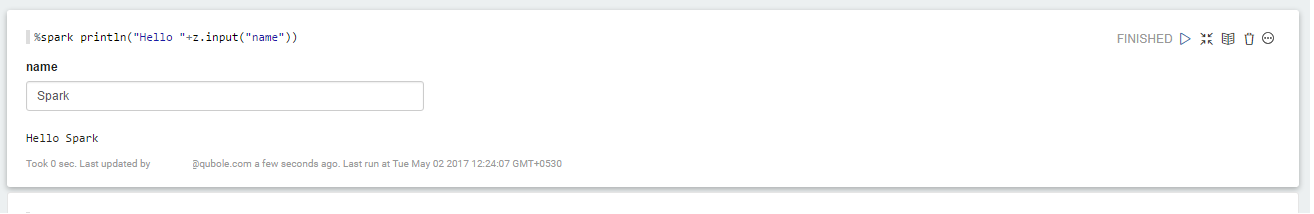A simple check box form can be created as shown in this example.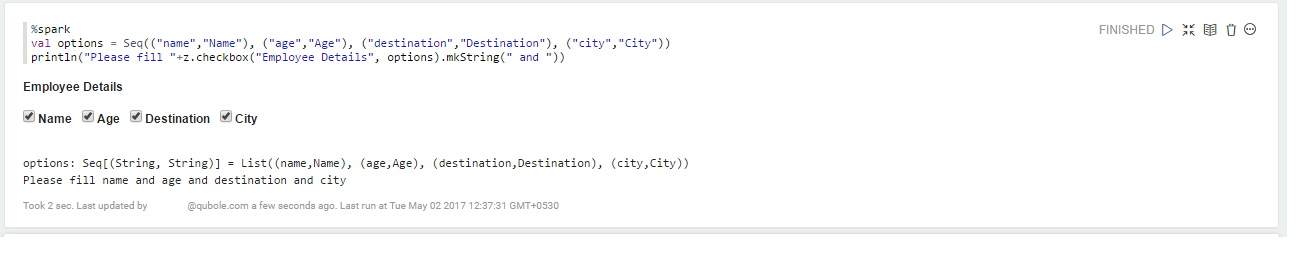If your cluster is running Zeppelin 0.8 or a later version, you can use notebook level dynamic input form. With the notebook level dynamic input form, you can use a single input form multiple times in multiple paragraphs.

The following table lists the parameters that you can use to create paragraph level and notebook level dynamic input forms.

Scope Paragraph Scope Notebook
\$ \$\$
z.input / z.textbox z.noteTextbox
z.select z.noteSelect
z.checkbox z.noteCheckbox

You can pass Zeppelin’s dynamic input variables to Shell and SQL interpreters by enabling the properties `zeppelin.shell.interpolation` and `zeppelin.spark.sql.interpolation` in shell and spark interpreter group from the Interpreters Settings page.

The following example shows how to pass variables to SQL interpreters.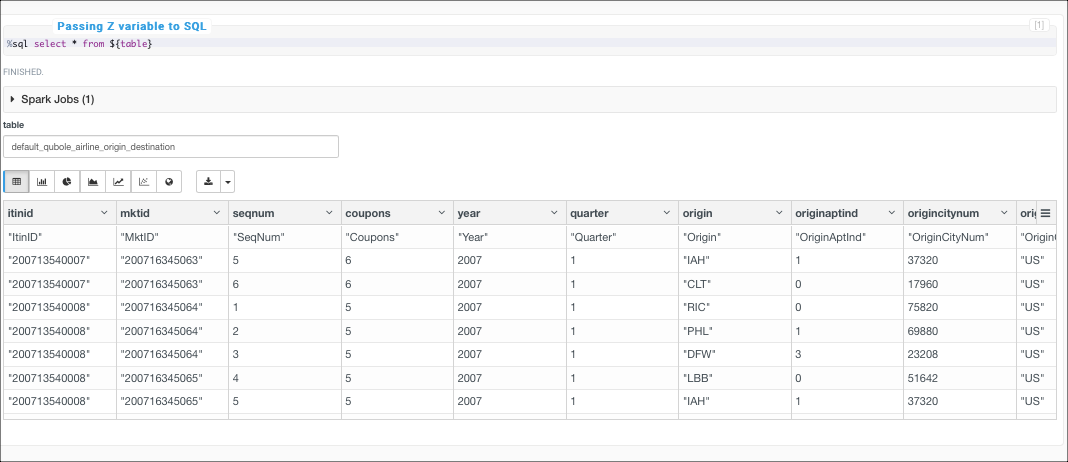## Example¶

The following example shows how to use `z.bind()` function to create universal variable with dynamic forms. You can run this sample code as a paragraph on the Notebooks page.

First Paragraph

```%spark

z.angularBind("year",z.input("Year"))
```

Second Paragraph

```%spark
z.show(sqlContext.sql("""
SELECT avg(depth) as avg_depth, max(depth) as max_depth, min(depth) as min_depth
FROM eq
WHERE year = """ + z.angular("year") + """
"""
))
```

Third Paragraph

```%spark
z.show(sqlContext.sql("""
SELECT avg(lat) as avg_lat, avg(lon) as avg_lon
FROM eq
WHERE year = """ + z.angular("year") + """
"""
))
```

The following figure shows the sample paragraphs that use universal variables using Angular Bind.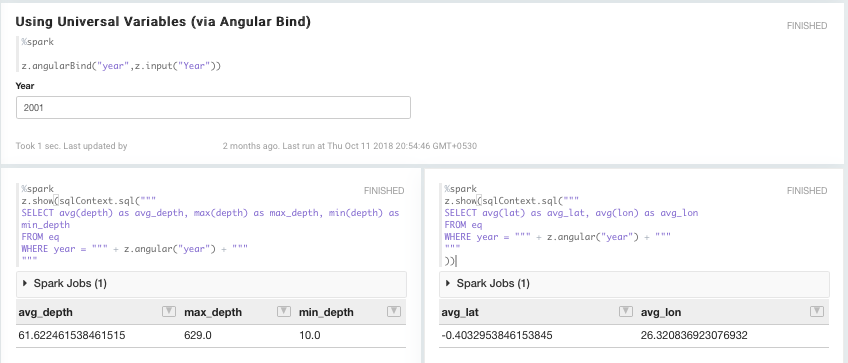The following animated GIF shows a sample notebook level dynamic input form.